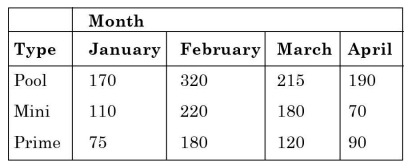Home » Verbal Ability » Numerical Ability » Question

#### Numerical Ability

1. Mola is a digital platform for taxis in a city. It offers three types of rides – Pool, Mini and Prime. The table below presents the number of rides for the past four months. The platform earns one US dollar per ride. What is the percentage share of revenue contributed by Prime to the total revenues of Mola, for the entire duration?1. 23.97
2. 16.24
3. 38.74
4. 25.86
##### Correct Option: A

Here,
Revenue shared by pool = 170 + 320 + 215 + 190 = 895\$
Similarly,
Revenue shared by mini = 110 + 220 + 180 + 70 = 580\$
and,
Revenue shared by prime = 75 + 180 + 120 + 90 = 465\$
Now, percentage share of revenue contributed by prime

 = 465 = 23.97% 895 + 580 + 465